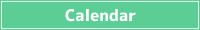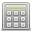<< < June 2023 > >>
SunMonTueWedThuFriSat
28 29 30 31 1 2 3
4 5 6 7 8 9 10
11 12 13 14 15 16 17
18 19 20 21 22 23 24
25 26 27 28 29 30 1## What is body surface area (BSA)?

BSA is an abbreviation for Body Surface Area, which is the surface area of the human body.

In the medical field, BSA is sometimes used to determine the dosage of drugs and to determine the extent of burns.

There are several calculation formulas, among which the Du Bois formula, the Shintani formula, and the Fujimoto formula are used.

## Formula for Body Surface Area (BSA)

BSA is calculated by the following formula.

### Du Bois formula

BSA(m2) = Height0.725(m) x Body weight0.425(kg) x 0.007184

### Shintani formula

BSA(m2) = Height0.725(m) x Body weight0.425(kg) x 0.007358

### Fujimoto formula

BSA(m2) = Height0.663(m) x Body weight0.444(kg) x 0.008883

###Simple Calculator

Save results in a temporary